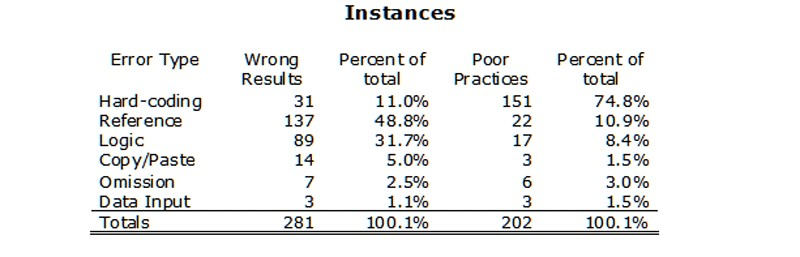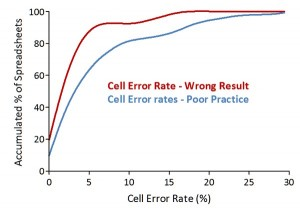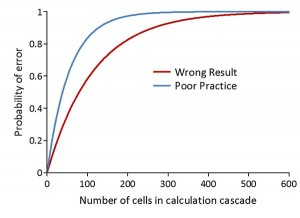# The Risk of Spreadsheet Errors

This entry is part 1 of 2 in the series Spreadsheet Errors

Spreadsheets create an illusion of orderliness, accuracy, and integrity. The tidy rows and columns of data, instant calculations, eerily invisible updating, and other features of these ubiquitous instruments contribute to this soothing impression.  The quote are taken from Ivars Peterson’s MathTrek Column written in back in 2005, but it still applies to day.1

Over the years we have learned a good deal about spreadsheet errors we even have got a spread sheet risk interest group (EuSpRIG)2.

Audits done shows that nearly 90% of the spreadsheets contained serious errors. Code inspection experiments also shows that even experienced users have a hard time finding errors succeeding in only finding 54% on average.

Panko (2009) summarized the results of seven field audits in which operational spreadsheets were examined, typically by an outsider to the organization. His results show that 94% of spreadsheets have errors and that the average cell error rates (the ratio of cells with errors to all cells with formulas) is 5.2%.3

Some of the problems stems from the fact that a cell can contain any of the following: operational values, document properties, file names, sheet names, file paths, external links, formulas, hidden cells, nested Ifs, macros etc. and that the workbook can contain, hidden sheets and very hidden sheets.

Add to this reuse and recirculation of workbooks and code; after cutting and pasting information, the spreadsheet might not work the way it did before — formulas can be damaged, links can be broken, or cells can be overwritten. How many uses version controls and change logs? In addition the spreadsheet is a perfect environment for perpetrating fraud due to the mixture of formulae and data.

End-users and organizations that rely on spreadsheets generally do not fully recognize the risks of spreadsheet errors:  It is completely within the realms of possibility that a single, large, complex but erroneous spreadsheet could directly cause the accidental loss of a corporation or institution (Croll 2005) 4

A very comprehensive literature review on empirical evidence of spreadsheet errors is given in the article Spreadsheet Accuracy Theory. 5

EUSPRIG also publicises verified public reports with a quantified error or documented impact of spreadsheet errors.6

We will in the following use publicised data from a well documented study on spreadsheet errors. The data is the result of an audit of 50 completed and operational spreadsheets from a wide variety of sources.7

Powell et alii settled for six error types:

1. Hard-coding in a formula – one or more numbers appear in formulas
2. Reference error – a formula contains one or more incorrect references to other cells
3. Logic error – a formula is used incorrectly, leading to an incorrect result
4. Copy/Paste error – a formula is wrong due to inaccurate use of copy/paste
5. Omission error – a formula is wrong because one or more of its input cells is blank
6. Data input error – an incorrect data input is used

And these were again grouped as Wrong Result or Poor Practise depending on the errors effect on the calculation.

Only three workbooks were without errors, giving a spreadsheet error rate of 94%. In the remaining 47 workbooks they found 483 instances8 of errors; 281 giving wrong result and 202 involving poor practise.The distribution on the different types of error is given in the instances table. It is worth noting that in poor practice hard-coding errors was the most common while incorrect references and incorrectly used formulas was the most numerous errors in wrong result.The 483 instances involved 4,855 error cells, which with 270,722 cells audited gives a cell error rate of 1.79%. The corresponding distribution of errors is given in the cells table. The Cell Error Rate (CER) for wrong result is 0.87% while the CER for poor practise is 1.79%.

In the following graph we have plotted the cell error rates against the proportion of spreadsheets having that error rate (zero CER is excluded). We can se that most spreadsheets have a low CER and only a few a high CER. This is more evident for wrong result than for poor practise.If we accumulate the above frequencies and include the spreadsheets with zero errors we get the “probability distributions” below. We find that 60% of the spread sheets have a CER giving a wrong result of 1% or more and that only 10% have a CER of 5% or more.The high percentage of spreadsheets having errors is due to the fact that bottom-line values are computed through long cascades of formula cells. Because in tasks that contain many sequential operations error rates multiply along cascades of subtasks, the fundamental equation for the bottom-line error rate is based on a memoryless geometric distribution over cell errors.9:Here, E is the bottom-line error rate, e is the cell error rate and n is the number of cells in the cascade. E indicates the probability of an incorrect result in the last cascade cell, given the probability of an error in each cascade cell is equal to the cell error rate.10

In the figure below we have used the CER for wrong result (0.87%) and for poor practise (1.79%) to calculate the probability of a corresponding worksheet error, given the cascade length. For poor practice at a calculation cascade of 100 cells there is a probability of 84% an error and 65 cells it is 95%. For wrong result 100 cells give a probability of 58% for an error and at 343 cells it is 95%.Now if we consider a net present value calculation over a 10 year forecast period in a valuation problem it will easily have more than 343 cells that with high probability contains error.

This is why S@R uses programming languages for simulation models. Of course will models like that also have errors, but it will not mix data and code, the quality control is easier, it will have columnar consistency, be protected by being compiled, having numerous intrinsic error checks, data entry controls and validation checks (see: Who we are).

Efficient computing tools are essential for statistical research, consulting, and teaching. Generic packages such as Excel are not sufficient even for the teaching of statistics, let alone for research and consulting (American Statistical Association )

### References

Series NavigationThe Most Costly Excel Error Ever? >>
1. Peterson, Ivars. “The Risky Business of Spreadsheet Errors.” MAA Online December 19, 2005 26 Feb 2009 . []
2. EuSpRIG: http://www.eusprig.org/index.htm []
3. Panko, Raymond R.. “What We Know About Spreadsheet Errors.” Spreadsheet Research (SSR. 2 16 2009. University of Hawai’i. 27 Feb 2009 . []
4. Croll, Grenville J.. “The Importance and Criticality of Spreadsheets in the City of London.” Notes from Eusprig 2005 Conference . 2005. EuSpRIG. 2 Mar 2009 . []
5. Kruck, S. E., Steven D. Sheetz. “Spreadsheet Accuracy Theory.” Journal of Information Systems Education 12(2007): 93-106. []
6. ” Spreadsheet mistakes – news stories.” EuSpRIG. 2 Mar 2009 . []
7. Powell, Stephen G., Kenneth R. Baker, Barry Lawson. “Errors in Operational Spreadsheets.” Tuck School of Business. November 15, 2007. Dartmouth College. 2 Mar 2009 []
8. An error instance is a single occurrence of one of the six errors in their taxonomy []
9. Lorge, Irving, Herbert Solomon. “Two Models of Group Behavior in the Solution of Eureka-Type Problems.” Psykometrika 20(1955): 139-148. []
10. Bregar, Andrej. “Complexity Metrics for Spreadsheet Models.” Proceedings ofEuSpRIG 2004. http://www.eusprig.org/. 1 Mar 2009 . []1.Ron Tedwater says: